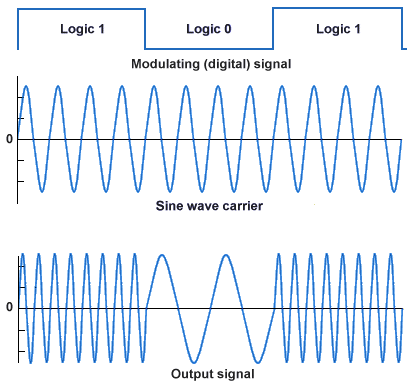# Frequency Shift Keying (FSK)

Frequency shift keying (FSK) is one of several techniques used to transmit a digital signal on an analogue transmission medium. The frequency of a sine wave carrier is shifted up or down to represent either a single binary value or a specific bit pattern. The simplest form of frequency shift keying is called binary frequency shift keying (BFSK), in which the binary logic values one and zero are represented by the carrier frequency being shifted above or below the centre frequency. In conventional BFSK systems, the higher frequency represents a logic high (one) and is referred to as the mark frequency. The lower frequency represents a logic low (zero) and is called the space frequency. The two frequencies are equi-distant from the centre frequency. A typical BFSK output waveform is shown below.Binary Frequency Shift Keying (BFSK)

If there is a discontinuity in phase when the frequency is shifted between the mark and space values, the form of frequency shift keying used is said to be non-coherent, otherwise it is said to be coherent. In more complex schemes, additional frequencies are used to enable more than one bit to be represented by each frequency used. This provides a higher data rate, but requires more bandwidth (representing a group of two binary values, for example, would require four different frequencies). It also increases the complexity of the modulator and demodulator circuitry, and increases the probability of transmission errors occurring.

## Audio frequency shift keying (AFSK)

Audio frequency-shift keying (AFSK) is a modulation technique in which binary data is represented by changes in the frequency of an audio tone, and is one of the techniques used for transmission on analogue telephone lines. Two tones are normally used to represent the mark and space values. Many early analogue modems employed AFSK to transmit data at rates of up to about 300 bits per second, and some early microcomputers used a modified form of AFSK to store data on audio cassettes.